# Bending moments and shearing forces in beams - singularity functions

In other tutorials the expressions for shearing forces and bending moments for different beam loading conditions are derived from equations for static equilibrium.  In this tutorial we demonstrate an alternative method for determining shearing forces V and bending moments M using singularity functions.

Singularity functions appear complex at first but once you understand the "rules" the method becomes clearer.

It must be understood that singularity functions are not normal algebraic expressions. They are conventionally enclosed within angle brackets < >.  Singularity functions apply at discontinuities, such as load points and changes in load conditions along a beam.  We define discontinuities occurring at  points a on the x axis (see below).  The singularity function is defined by the expression <x - a>n where the exponent n can be -ve, +ve or 0.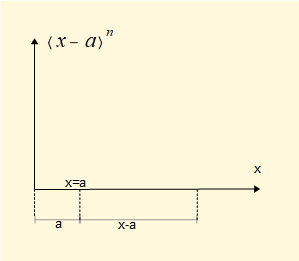There are two sets of rules concerning singularity functions that we use:

• values of the function when x < a, x = a and x > a
• integrals of the function for specific values of the exponent n

These properties are defined below for the range of functions (i.e. exponents) of interest to us. Note that a specific type of applied load is associated with each function. Note also the formal generic name of each function (e.g. unit impulse).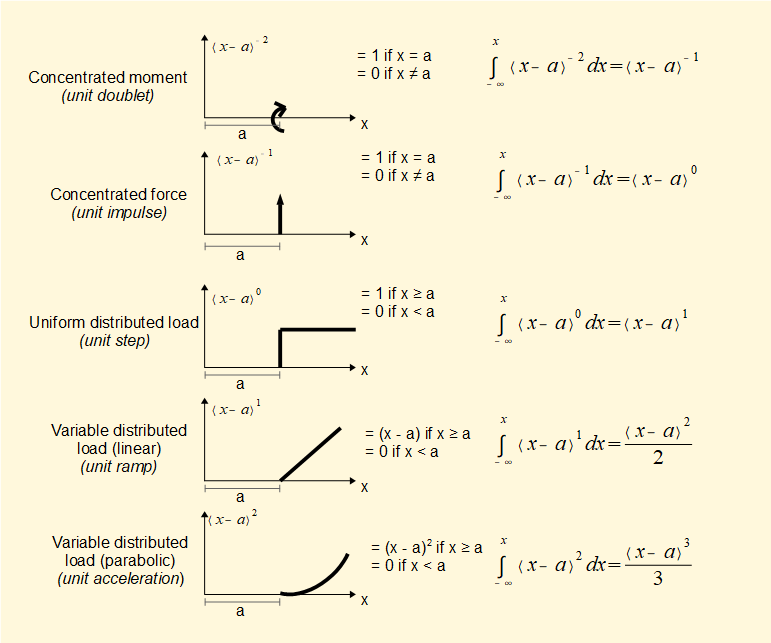We now define a loading function q(x) corresponding to each singularity function as follows:

• for an applied moment M0 (kNm) at x = a    q(x) = M0<x - a>-2

•  for a concentrated force F (kN) at x = a    q(x) = -F<x - a>-1

•  for a uniformly distributed load w (kN/m) applied from x = a    q(x) = -w<x - a>0

• for a linear variable distributed load s (kN/m2) applied from x = a    q(x) = -s<x - a>1

Note that the signs for the above functions are valid for positive (clockwise) applied moments and negative applied forces and must be reversed where necessary (the example below will demonstrate this).

A loading function q comprising all moments and loads applied to the beam is then stated and expressions for V and M obtained using the following relationships.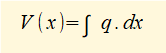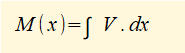We now show an example of the method using a simply supported beam with two point loads and a uniformly distributed load over a portion of the beam.Note the equivalent point load of distributed load w is 30 kN with centre of action at x = 4.5 m

To find R1 and R2:

(a) sum of forces = 0   (R1 and R2 are +ve forces; F1, F2, and w are -ve forces)

R1 + R2 = F1 + F2 + 3w  ------(i)

(b) sum of moments about x(0) = 0  (clockwise moments are +ve)

(6 x R2) = (30 x 4.5) + (5 x 3) + (20 x 1.5 ) = 135 + 15 + 30 = 180

Thus    R2 = (180)(/6) = 30 kN

From (i)     R1 = F1 + F2 + 3 w - R2 = 20 + 5 + 30 - 30 = 25

Thus     R1 = 25 kN

We now state the loading function q(x) for each load element in terms of the singularity function <x - a>n   where a is the value of x corresponding to the location of the point loads or the initial point of loading for the distributed load (as x increases along the beam).

For R1    q(x) = R1<x - 0>-1    (note R1 acts in the +ve direction - see comment above regarding sign)

For F1    q(x) = -F1<x - 1.5>-1

For F2    q(x) = -F2<x - 3>-1

For w      q(x) = -w<x - 3>0        (note a = 3  is the initial point of loading for the distributed load)

For R2    q(x) = R2<x - 6>-1

q(x) =   R1<x - 0>-1  -F1<x - 1.5>-1  -F2<x - 3>-1  -w<x - 3>0  + R2<x - 6>-1Thus       V =  R1<x - 0>0 -F1<x - 1.5>0  -F2<x - 3>0  -w<x - 3>1  + R2<x - 6>0

Now we evaluate each singularity function using the rules defined above for x = a.  Remember the value of all the singularity functions = 0 for x < a

Gives      <x - 0>0 = 1     <x - 1.5>0  = 1      <x - 3>0  = 1      <x - 3>1 = (x - 3)    <x - 6>0  = 1

note the transformation of  <x - 3>1 to the algebraic function (x - 3)

These expressions translate to values of V as follows:

At x = 0 there is a step change of V = R1 = 25 kN

Thus from x = 0 to x = 1.5    V = R1 = 25 kN

At x = 1.5 there is a step change of V = -F1 = -20 kN

Thus from x = 1.5 to x = 3    V = (25 - F1) = (25 - 20) = 5 kN

At x = 3 there is a step change of V = -F2 = -5 kN and a linear function V = -w(x - 3) =  -10(x - 3) kN

Thus from  x = 3 to x = 6        V = [5 - (F2) - w(x - 3)] = [(5 - 5 -10(x - 3)] = (30 - 10x) kN

At x = 6   there is a step change of V = R2 = 30 kN

Thus at x = 6      V = [(30 - 10x) + R2] = [30 - 10x +30] = 0 (by substituting x = 6).  Note this final step simply acts as a check as we already know V = 0 at x = 6.

The shearing force diagram is shown below.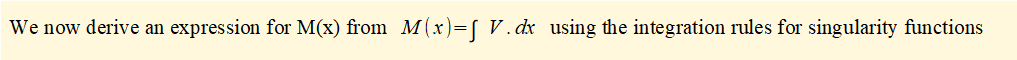From above    V =  R1<x - 0>0 -F1<x - 1.5>0  -F2<x - 3>0  -w<x - 3>1  + R2<x - 6>0

Thus       M =  R1<x - 0>1 -F1<x - 1.5>1  -F2<x - 3>1  -w<x - 3>2  + R2<x - 6>1

Again we evaluate the singularity functions using the stated rules for x = a

<x - 0>1 = x     <x - 1.5>1  = (x - 1.5)      <x - 3>1  = (x - 3)      <x - 3>2 = (x - 3)2/2     <x - 6>1  = (x - 6)

note that all expressions are transformed to algebraic functions of x

These expressions translate to values of M (kNm) as follows:

From x = 0 to x = 1.5    there is a linear function M = (R1)x = 25x

From x = 1.5 to x = 3    there is a linear function M = [(25x} - F1(x - 1.5)] = [(25x) - 20(x - 1.5)] = (5x + 30)

From x = 3 to x = 6    there is a quadratic function M = (5x + 30) - F2(x - 3) - w(x - 3)2/2

= 5x +30 - 5(x - 3) - 10(x - 3)2/2

= [5x + 30 - 5x + 15 - 5x2 +30x - 45] = [30x - 5 x2 ]

= 5(6x - x2 )

At x = 6 the function (R2} (x - 6) = 30(x - 6) applies but  this function makes no tangible contribution to the bending moment as non-zero values are beyond the physical extent of the beam.

The bending moment diagram is shown below.In summary, with singularity functions we have a very powerful method for determining V and M for different loading combinations.

I welcome feedback at:

### Tutorials - mechanical vibrations

###### Forced vibrations with damping

Alistair's tutorials 2019